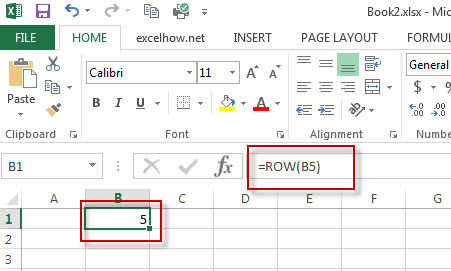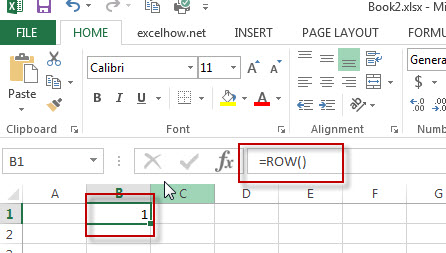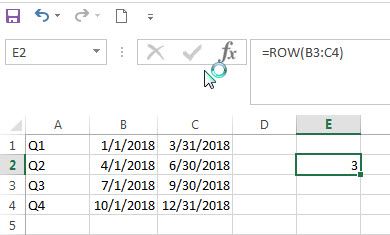Excel Row Function

This post will guide you how to use Excel ROW function with syntax and examples in Microsoft excel.

Description

The Excel ROW function returns the row number of a cell reference.

The ROW function is a build-in function in Microsoft Excel and it is categorized as a Lookup and Reference Function.

The ROW function is available in Excel 2016, Excel 2013, Excel 2010, Excel 2007, Excel 2003, Excel XP, Excel 2000, Excel 2011 for Mac.

Syntax

The syntax of the ROW function is as below:

= ROW ([reference])

Where the ROW function arguments is:
Reference – This is an Optional argument. the cell or range of cells reference that you want to get the row number.

Note: If the reference is omitted, the ROW function will return the row number of the current cell.

Excel Row Function Examples

The below examples will show you how to use Excel ROW Lookup and Reference Function to return the row number of a cell reference.
#1 To get the row number of “B5” cell in B1 Cell, just using the following excel formula: =ROW(B5)#2 To get the row number of the current cell, just using excel formula: =ROW()#3 get the first row number of range in excel

You can get the first row number in a range with an excel formula based on the ROW function as follows:

=ROW(Range)
=MIN(ROW(Range))

The ROW function will only display the first row number.Related Functions

• Excel MIN function
The Excel MIN function returns the smallest numeric value from the numbers that you provided. Or returns the smallest value in the array.The MIN function is a build-in function in Microsoft Excel and it is categorized as a Statistical Function.The syntax of the MIN function is as below:= MIN(num1,[num2,…numn])….

More Excel ROW Formula Examples

• Get the Position of the nth Occurrence of a Character in a Cell
If you want to get the position of the nth occurrence of a character using a excel formula, you can use the FIND function in combination with the SUBSTITUTE function.
•  Get the position of Last Occurrence of a character or string in a cell
If you want to get the position of the last occurrence of a character in a cell, then you can use a combination of the LOOKUP function, the MID function, the ROW function, the INDIRECT function and the LEN function to create an excel formula.…
• Find the Relative Position in a Range or Table
If you want to know the relative row position for all rows in an Excel Range (B3:D6), you can use a excel Array formula as follows:=ROW(B3:D6)- ROW(B3) + 1. You can also use another excel array formula to get the same result as follows:=ROW(B3:D6)-ROW(INDEX(B3:D6,1,1))+1…
• Get the First Row Number in a Range
If the ROW function use a Range as its argument, it only returns the first row number.You can also use the ROW function within the MIN function to get the first row number in a range. You can also use the INDEX function to get the reference of the first row in a range, then combined to the ROW function to get the first row number of a range.…
•  Get the Last Row Number in a Range
If you want to get the last row number in a range, you need to know the first row number and the total rows number of a range, then perform the addition operation, then subtract 1, the last result is the last row number for that range.…
• Get the position of Last Occurrence of a value in a column
If you want to find the position number of the last occurrence of a specific value in a column (a single range), you can use an array formula with a combination of the MAX function, IF function, ROW function and INDEX Function.
• Get the position of nth occurrence of a value in column
If you want to locate the second, third or nth occurrence of a specified value, you can use a combination of the INDEX function, the IF function, ROW function and SMALL function to create a new excel array formula.
• Split Text String to an Array
If you want to convert a text string into an array that split each character in text as an element, you can use an excel formula to achieve this result. the below will guide you how to use a combination of the MID function, the ROW function, the INDIRECT function and the LEN function to split a string…
• Three Ways to Get the Last Row Number in a Range
If you want to get the last row number in a range, you need to know the first row number and the total rows number of a range, then perform the addition operation, then subtract 1, the last result is the last row number for that range…
• Three Ways to get the First Row Number in a Range
you can use ROW function to get the first row number in a range directly. You can also use the ROW function within the MIN function to get the first row number in a range.You can also use the INDEX function to get the reference of the first row in a range, then combined to the ROW function to get the first row number of a range…
• Transpose Multiple Columns into One Column
You can use the following excel formula to transpose multiple columns that contain a range of data into a single column, and you can also write an Excel VBA Macro to transpose the data of range in B1:D4 into single column F quickly…
• Delete Every Other Row
To delete every other row in Excel, you need to filter alternate rows, and then select those rows, and delete them at once. So you need to create a helper column using MOD function with ROW function…
• Sum Numbers from 1 to N
Assuming that you supply a number 100, and you want to sum the numbers from 1 to 100 (1+2+3+…+100), you need to create a formula based on the SUMPRODUCT function, the ROW function and the INDIRECT function to achieve the result..….
• Split Data in One Column to Multiple Columns
If you want to split each sets in one column from row to row in stacked columns, and you need to create a formula based on the OFFSET function, the COLUMNS function, and the ROWS function..…
• VLOOKUP Return Multiple Values Horizontally
You can create a complex array formula based on the INDEX function, the SMALL function, the IF function, the ROW function and the COLUMN function to vlookup a value and then return multiple corresponding values horizontally in Excel.…
• Copy and Paste Only Non-blank Cells
If you want only copy non-blank cells in a range in Excel, you need to select the non-blank cells firstly, then press Ctrl +C keys to copy the selected cells. So how to only select all non-blank cells in the selected range in your worksheet..…
• Sum Every Nth Row or Column
If you want to sum every nth rows in Excel, you can create an Excel Array formula based on the SUM function, the MOD function and the ROW function..….
• Generate All Possible Combinations of Two Lists
You can use a formula based on the IF function, the ROW function, the COUNTA function, The INDEX function and the MOD function to get a list of all possible combinations from those two list….
• Find Missing Numbers in a Sequence in Excel
You can use an excel array formula based on the SMALL function, the IF function, the ISNA function, the MATCH function, and the ROW function to find missing numbers in a sequence…

Related Posts

Average the Last N Numeric Values in Excel

AVERAGE function is one of the most popular functions in Excel. Apply AVERAGE together with some other functions, we can calculate average simply for some complex situations. In this article, we will introduce you to calculate average of the last ...

How To Sum the Largest N Values in Excel

Sometimes we may want to sum the largest N numbers or top N numbers in a range. In this article, we will show you the method of “SUM the Largest N Numbers” by a simple formula which consist of SUMPRODUCT ...

How to Sum the Smallest N Values in Excel

Sometimes we may want to sum the first smallest N numbers in a range in Excel. In this article, we will show you the method of “SUM the Smallest N Numbers” by a simple formula which consist of SUMPRODUCT and ...

How to Auto Fill Weekdays or Weekends in Excel

Sometimes we may want to enter a sequence of days in excel for some purpose, and we can press Ctrl+; to insert current date into cell, and then drag the cell down to attach following days into other cells, then ...

How to Create Dynamical Drop-Down List and Sort by Alphabetical Order in Excel

In our daily work we may need to create a dynamical dropdown list and sort all values by alphabetical order. To create a dropdown list like this, we need to apply some built-in features like ‘Define Name’ and ‘Data Validation’ ...

How to Highlight Every Other Row or Every Nth Row in Excel?

Sometimes we may want to highlight every other row or every Nth row in a spreadsheet to make data in different rows looks more clearly. Though we can apply table styles to highlight table rows automatically, we can also highlight ...

How to Count Duplicate Values Only Once in A Range in Excel?

When counting the number of times for objects appear in a list or a range, we usually record the duplicate value only once and ignore the redundant ones. We cannot apply formula with only one function to implement this in ...

How to Create Dynamic Drop Down List without Blank in Excel

This post will guide you how to create dynamic drop down list without blank cells in Microsoft Excel. In Excel, and you can use Data Validation feature to improve the efficiency of data entry in excel, and it also be ...

How to Stack Data from Multiple Columns into One Column in Excel

In previous article, I have shown you the method to split data from one long column to multiple columns by VBA and Index function. This time if we want to stack data from multiple columns to one column, how can ...

How to Count Only Unique Values Excluding Duplicates in Excel

We enter a list of numbers or products and there are some duplicates in the list, if we want to just do count for the unique values and exclude the duplicates, how can we do? Now you can follow the ...

Sidebar